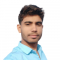## The Realization of Logic Functions Using NAND GatesUpdated on 10-Jan-2023 11:20:03
A logic function is a basically a Boolean expression that returns a Boolean value, which is a value that is either TRUE or FALSE. The NAND gate is a universal logic gate, thus it can be used to implement any type of logic function. Read this tutorial to learn how you can realize different logic functions using NAND gates. Let's start with a basic introduction of the NAND gates What is a NAND Gate? A NAND Gate is a type of universal logic gate that one can use to realize any kind logical expression or any other type of logic ... Read More

## Implementation of XOR Gate from NAND GateUpdated on 10-Jan-2023 11:07:48
The NAND Gate is a universal logic gate, using which we can implement any other type of logic gate or logical expression. Read this tutorial to understand how you can implement a XOR gate using only NAND gates. Let's start with a basic overview of XOR and NAND gates What is a XOR Gate? The XOR (Exclusive-OR) Gate is a type of derived logic gate. The XOR gate is a logic gate that has two inputs and one output. The XOR gate produces a HIGH (Logic 1) output when one and only one of its two inputs are HIGH (Logic ... Read More

## Implementation of XNOR Gate from NAND GateUpdated on 10-Jan-2023 11:04:54
As we know, the NAND Gate is a universal logic gate, using which we can implement any other type of logic gate or logical expression. Read this tutorial to find out how you can implement an XNOR gate using only NAND gates. Let's start with a basic overview of XNOR and NAND gates. What is an XNOR Gate? The XNOR (Exclusive-NOR) Gate is a type of derived logic gate. The XNOR gate is a logic gate that has two inputs and one output. The XNOR gate produces a HIGH (Logic 1) output when both of its inputs are equal, i.e. ... Read More

## Implementation of OR Gate from NAND GateUpdated on 10-Jan-2023 10:58:09
NAND Gate is a universal logic gate, using which we can realize any logic gate. Read this tutorial to find out how you can realize an OR gate using a NAND gate. Before going into the implementation part, let's have a brief overview of OR and NAND gates. What is an OR Gate? An OR Gate is a basic logic gate. An OR gate may accept two or more than two inputs, but gives only one output. The OR gate gives a HIGH (Logic 1) output if any one of its inputs is in the HIGH or Logic 1 state, ... Read More

## Implementation of NOR Gate from NAND GateUpdated on 10-Jan-2023 10:52:26
NOR and NAND gates are universal logic gates, using which we can implement any logic gate or any other logical expression. Read this tutorial to find out how you can implement a NOR gate using a NAND gate. What is a NOR Gate? NOR Gate is a type of universal logic gate, because this logic gate can be used for implementation of any other type of logic gate. NOR means "NOT + OR". That means the OR output is NOTed or inverted. Therefore, the NOR gate is a combination of OR gate and a NOT gate. $$\mathrm{NOR\:Gate = OR\:Gate \:+\:NOT\:Gate}$$ ... Read More

## Implementation of AND Gate from NAND GateUpdated on 02-Feb-2023 15:04:47
As we know that the NAND Gate is a universal logic gate, therefore using the NAND gate, we can implement any logic gate or any other logical expression. Read this tutorial to understand how you can implement an AND gate using NAND gate. Let's start with a basic overview of AND and NAND gates. What is an AND Gate? An AND Gate is a basic logic gate. An AND gate may have two or more than two inputs, but gives only one output. The AND gate gives a LOW (Logic 0) output if any one of its inputs is in ... Read More

## Realization of a Full Subtractor using NAND gateUpdated on 26-Dec-2022 18:30:18
In digital electronics, a subtractor is a combinational logic circuit that performs the subtraction of two binary numbers. However, the subtraction of binary number can be performed using adder circuits by taking 1s or 2s compliments. But, we may also realize a dedicate circuit to perform the subtraction of two binary numbers. In the subtraction of two binary numbers, each subtrahend bit of the number is subtracted from its corresponding significant minuend bit to form a difference bit. During the subtraction, if the minuend bit is smaller than the subtrahend bit, then a 1 is borrowed from the next position. ... Read More

## Implementation of NOT Gate using NAND GateUpdated on 26-Dec-2022 18:17:26
Before getting into implementing a NOT gate using NAND gate, let’s have a basic overview of NOT gates and NAND gates. What is NOT Gate? NOT gate is a basic logic gate used in digital electronic circuits. The NOT gate has a single input and a single output. The output of the NOT gate is the logical inversion of its input. For this reason, the NOT gate is also known as inverter. The symbol of the standard NOT gate has a triangle pointing to the right with a circle at its right end as shown in figure-1. This circle ... Read More